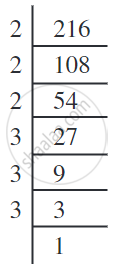# Some Interesting Patterns of Cube Numbers:

## 1. Adding consecutive odd numbers:

1 = 1 = 13
3 + 5 = 8 = 23
7 + 9 + 11 = 27 = 33
13 + 15 + 17 + 19 = 64 = 43
21 + 23 + 25 + 27 + 29 = 125 = 53

We can see from the above pattern,
If we want n3, then we needed n consecutive odd numbers such that the sum of consecutive odd numbers is equal to n3.

## 2. Cubes and their prime factors:

Consider the following prime factorisation of the numbers and their cubes.

 Prime factorisation of a number Prime factorisation of its cube 4 = 2 × 2 43 = 64 = 2 × 2 × 2 × 2 × 2 × 2 = 23 × 23. 6 = 2 × 3 63 = 216 = 2 × 2 × 2 × 3 × 3 × 3 = 23 × 33. 15 = 3 × 5 153 = 3375 = 3 × 3 × 3 × 5 × 5 × 5 = 33 × 53. 12 = 2 × 2 × 3 123 = 1728 = 2 × 2 × 2 × 2 × 2 × 2 × 3 × 3 × 3 = 23 × 23 × 33.

Observe that each prime factor of a number appears three times in the prime factorisation of its cube.

If in the prime factorisation of any number each factor appears three times, then the number is a perfect cube.

1) Is 216 a perfect cube?By prime factorisation, 216 = 2 × 2 × 2 × 3 × 3 × 3....(factors can be grouped in triples)

Each factor appears 3 times.

216 = 23 × 33 = (2 × 3)3 = 63 which is a perfect cube.

## 3) Smallest multiple that is a perfect cube

1) Is 392 a perfect cube? If not, find the smallest natural number by which 392 must be multiplied so that the product is a perfect cube.

Solution:
392 = 2 × 2 × 2 × 7 × 7
The prime factor 7 does not appear in a group of three. Therefore, 392 is not a perfect cube. To make its a cube, we need one more 7. In that case
392 × 7 = 2 × 2 × 2 × 7 × 7 × 7 = 2744 which is a perfect cube.

Hence the smallest natural number by which 392 should be multiplied to make a perfect cube is 7.

#### Example

Is 243 a perfect cube?

243 = 3 × 3 × 3 × 3 × 3

In the above factorisation 3 × 3 remains after grouping the 3’s in triplets.

Therefore, 243 is not a perfect cube.

#### Example

Is 53240 a perfect cube? If not, then by which smallest natural number should 53240 be divided so that the quotient is a perfect cube?
53240 = 2 × 2 × 2 × 11 × 11 × 11 × 5
The prime factor 5 does not appear in a group of three. So, 53240 is not a perfect cube. In the factorisation 5 appears only one time. If we divide the number by 5, then the prime factorisation of the quotient will not contain 5.
So, 53240 ÷ 5 = 2 × 2 × 2 × 11 × 11 × 11
Hence the smallest number by which 53240 should be divided to make it a perfect
cube is 5.
The perfect cube, in that case, is = 10648.

#### Example

Is 1188 a perfect cube? If not, by which smallest natural number should 1188 be divided so that the quotient is a perfect cube?
1188 = 2 × 2 × 3 × 3 × 3 × 11
The primes 2 and 11 do not appear in groups of three. So, 1188 is not a perfect cube. In the factorisation of 1188, prime 2 appears only two times and the prime 11 appears once. So, if we divide 1188 by 2 × 2 × 11 = 44, then the prime factorisation of the quotient will not contain 2 and 11.
Hence the smallest natural number by which 1188 should be divided to make it a
a perfect cube is 44.
And the resulting perfect cube is 1188 ÷ 44 = 27 (= 33).

#### Example

Is 68600 a perfect cube? If not, find the smallest number by which 68600 must be multiplied to get a perfect cube.
We have, 68600 = 2 × 2 × 2 × 5 × 5 × 7 × 7 × 7.
In this factorisation, we find that there is no triplet of 5.
So, 68600 is not a perfect cube.
To make it a perfect cube we multiply it by 5.
Thus,
68600 × 5 = 2 × 2 × 2 × 5 × 5 × 5 × 7 × 7 × 7
= 343000, which is a perfect cube.
If you would like to contribute notes or other learning material, please submit them using the button below.

### Shaalaa.com

Patterns of Cube Numbers - Part 1 [00:08:59]
S
0%